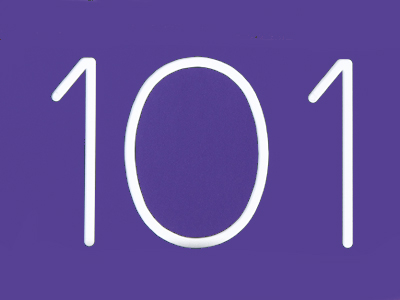101 centimetres is the same as 1.01 metres.

# Decimal Numbers 2 (Difficult)

Decimal numbers are the basis of metric measurements. They make calculations so much easier when converting from centimetres to metres, or from kilograms to grams for example. That’s because larger metric units are divided into tens, hundreds or thousands of smaller ones.

This series of quizzes is a little more difficult than those before it. However, by now you should have a very good grasp of decimal numbers, so you’ll be more than capable of answering these questions. If you are in any doubt, then go back and revisit our easier 11+ maths quizzes to get more practice.

Most of the questions you’ll find here concentrate on converting one measurement into another – something you’ll be called on to do often in real life. So, the more you familiarise yourself with doing it, the better placed you’ll be.

1.
How many kilograms are there in 2.3 tonnes?
2,300,000
23,000
230
2,300
There are 1,000 kg in 1 tonne, so in 2.3 tonnes there are 1,000 × 2.3 = 2,300 kg
2.
A gold bar weighs ‪12,442.61‬ grams. How many kilograms does a gold bar weigh?
1.244261 kg
1,244.261 kg
124.4261 kg
12.44261 kg
There are 1,000 g in 1 kg, so in 12,442.61 g there are 12,442.61 ÷ 1,000 = 12.44261 kg
3.
What was 9.87654 multiplied by if the answer is 987.654?
100
10
1,000
10,000
The rule for multiplying by a whole number beginning with 1 and followed by one or more zeros, e.g. multiplying by 10, 100, 1,000, ... is as follows: When multiplying by such a number, move the digits to the left by the same number of zeros as in the number: 100 × 9.87654 = 987.654: the digits have moved two places to the left = the number of zeros in one hundred
4.
What is 21.63 kilometres in centimetres?
2,163 cm
21,630 cm
2,163,000 cm
216,300 cm
Convert km to m by multiplying by 1,000. So, 21.63 x 1,000 = 21,630
Next, convert m to cm by multiplying by 100. So, 21,630 x 100 = 2,163,000
5.
What does 0.00025 have to be multiplied by to give 2.5?
1,000
10,000
100,000
100
For 0.00025 to become 2.5, the digits will have to move 4 places to the left. You will have to multiply 0.00025 by 10,000 - which has 4 zeros
6.
How many square centimetres are there in 15.3 square metres?
1,530 cm²
15,300 cm²
153,000 cm²
1,530,000 cm²
Be careful of squares! There are 100 cm in 1 m, so in 1 sq m there are 100 × 100 = 10,000 sq cm. In 15.3 sq m there are 15.3 × 10,000 = 153,000 sq cm
7.
What was 987.654 divided by if the answer is 0.987654?
1,000
100
10,000
10
The rule for dividing by a whole number beginning with 1 and followed by one or more zeros, e.g. dividing by 10, 100, 1,000, ... is as follows: When dividing by such a number, move the digits to the right by the same number of zeros as in the number: 987.654 ÷ 1,000 = 0.987654: the digits have moved three places to the right = the number of zeros in one thousand
8.
How many kilograms are there in 7,531.09 grams?
0.753109 kg
7.53109 kg
75.3109 kg
753.109 kg
There are 1,000 g in 1 kg, so in 7,531.09 g there are 7,531.09 ÷ 1,000 = 7.53109 kg
9.
The marathon is a race over a distance of 42.195 kilometres. How many metres is that?
4,219,500 m
421,950 m
42,195 m
4,219.5 m
There are 1000 m in 1 km, so in 42.195 km there are 227.65 × 1,000 = 42,195 m
10.
What is 14,253 centimetres in metres?
1,425.3 m
1.4253 m
14.253 m
142.53 m
There are 100 cm in 1 m, so in 14,253 cm there are 14,253 ÷ 100 = 142.53 m
Author:  Frank Evans